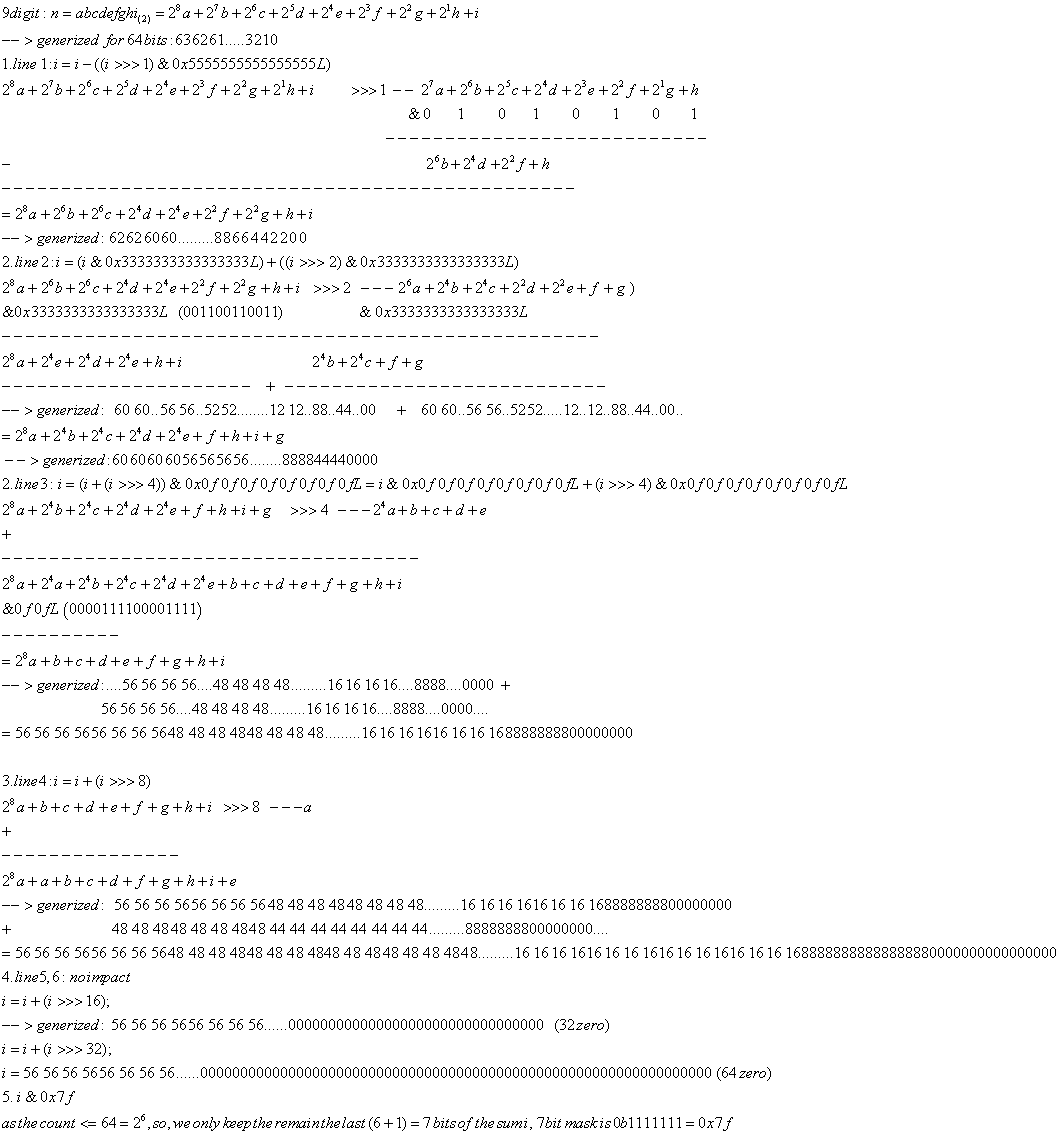# Java bitCount algorithm explanation

It is not strange to have bit wise operator and Left/Right Shift in a function. But it is definitely weird to have all statements with them in a function.  Yeah, maybe you think it is not true in the real production. You are wrong then. It exists in the Java SDK.  Below is copied of Long.bitCount function.

public static int bitCount(long i){

i = i – ((i  > > > 1) & 0x5555555555555555L);

i = (i & 0x3333333333333333L) + ((i  > > > 2) & 0x3333333333333333L);

i = (i + (i  > > > 4)) & 0x0f0f0f0f0f0f0f0fL;

i = i + (i  > > > 8);

i = i + (i  > > > 16);

i = i + (i  > > > 32);

return (int)i & 0x7f;

}

Ok. this article just uses a picture described the above function. Of course, it is a big picture. The function is to count how many 1 in a long integer. For example, bitCount(8)=1, as 8 is 1000 in binary. The algorithm in the function is to get the sum of each bit. As example of integer 8, it will be 1+0+0+0=1.  That is it. If you have a integer is abcdefgh in binary. The count will be a+b+c+d+e+f+g+h. For a 64bit integer, you need to get the sum of each bit.  The algorithm is to improve a lot performance of 1’s bit count in a long integer, comparing the naive loop to count.« (Previous News)